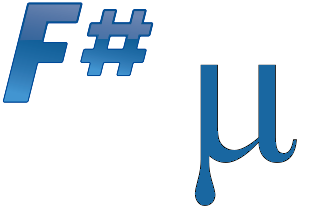# F# Fuzzy Library

Documentation

The F# Fuzzy library can be installed from NuGet:
PM> Install-Package FSharp.Fuzzy## Example

Fuzzy valuation of 2-year bond with 10% coupon.

  1: 2: 3: 4: 5: 6: 7: 8: 9: 10:  #r "FSharp.Fuzzy.dll" open FSharp.Fuzzy let i1 = Fuzzy.number(0.0011m,0.0012m,0.0014m) let i2 = Fuzzy.number(0.0008m,0.0011m,0.0016m) let M = 1000m let couponRate = 0.1m let coupon = M * couponRate let presentValue = coupon/(1m + i1) + (coupon + M)/Fuzzy.pow(1m + i2, 2.)

## Samples & documentation

The library comes with documentation.

The project is hosted on GitHub where you can report issues, fork the project and submit pull requests. If you're adding new public API, please also consider adding samples that can be turned into a documentation. You might also want to read library design notes to understand how it works.

The library is available under Public Domain license, which allows modification and redistribution for both commercial and non-commercial purposes. For more information see the License file in the GitHub repository.

namespace FSharp
namespace FSharp.Fuzzy
val i1 : Fuzzy

Full name: Index.i1
Multiple items
module Fuzzy

from FSharp.Fuzzy

--------------------
type Fuzzy =
interface IComparable
new : a:seq<Interval> -> Fuzzy
override Equals : yobj:obj -> bool
override GetHashCode : unit -> int
override ToString : unit -> string
member Bottom : Interval
member Top : Interval
member alphaCuts : Interval []
static member Zero : Fuzzy
static member map : f:(Interval -> Interval) -> a:Fuzzy -> Fuzzy
...

Full name: FSharp.Fuzzy.Fuzzy

--------------------
new : a:seq<Interval> -> Fuzzy
val number : a:decimal * b:decimal * c:decimal -> Fuzzy

Full name: FSharp.Fuzzy.Fuzzy.number
val i2 : Fuzzy

Full name: Index.i2
val M : decimal

Full name: Index.M
val couponRate : decimal

Full name: Index.couponRate
val coupon : decimal

Full name: Index.coupon
val presentValue : Fuzzy

Full name: Index.presentValue
static member Fuzzy.pow : x:Fuzzy * p:double -> Fuzzy